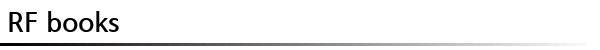조회수 : 2046 도서명 Introduction to the Uniform Geometrical Theory of Diffraction 저자/역자 Derek A. McNamara 출판사 Artech House 분야 전자파 수치해석 목차/설명 This book shows you how to solve high-frequency electromagnetic problems for which exact solutions of Maxwells equations are not possible. The authors discuss the basic concepts and ideas and then explain each diffraction phenomenon in turn. They offer teaching-based examples that guide you in applying these principles to real-world design and analysis problems. Designed for both self-study and guided instruction, Introduction to the Uniform Geometrical Theory of Diffraction includes only problem, established techniques. Over 1500 equations and 184 line drawings illustrate diffraction methods for solving typical radiation and scattering problems. Contents: Foreword. Preface. The Nature of High-Frequency Methods. Geometrical Optics Fields. Geometrical Optics Reflect Fields. Two-Dimensional Wedge Diffraction. Applications of Two-Dimensional Wedge Diffraction. Three-Dimensional Wedge Diffraction and Corner Diffraction. Equivalent Currents. Diffraction at a Smooth Convex Conducting Surface. Appendices. Index. 등록자 admin   수정하기
 ▼ 추천/비평(0)
 목록으로 이 책을 보신 분이라면 목차/ 내용추가설명이나 비평,추천/비추천에 대한 의사를 표시해주세요! 해당서적과 관련된 내용이면 어떤 내용이든 상관없습니다. 아이디 :    비밀번호 :